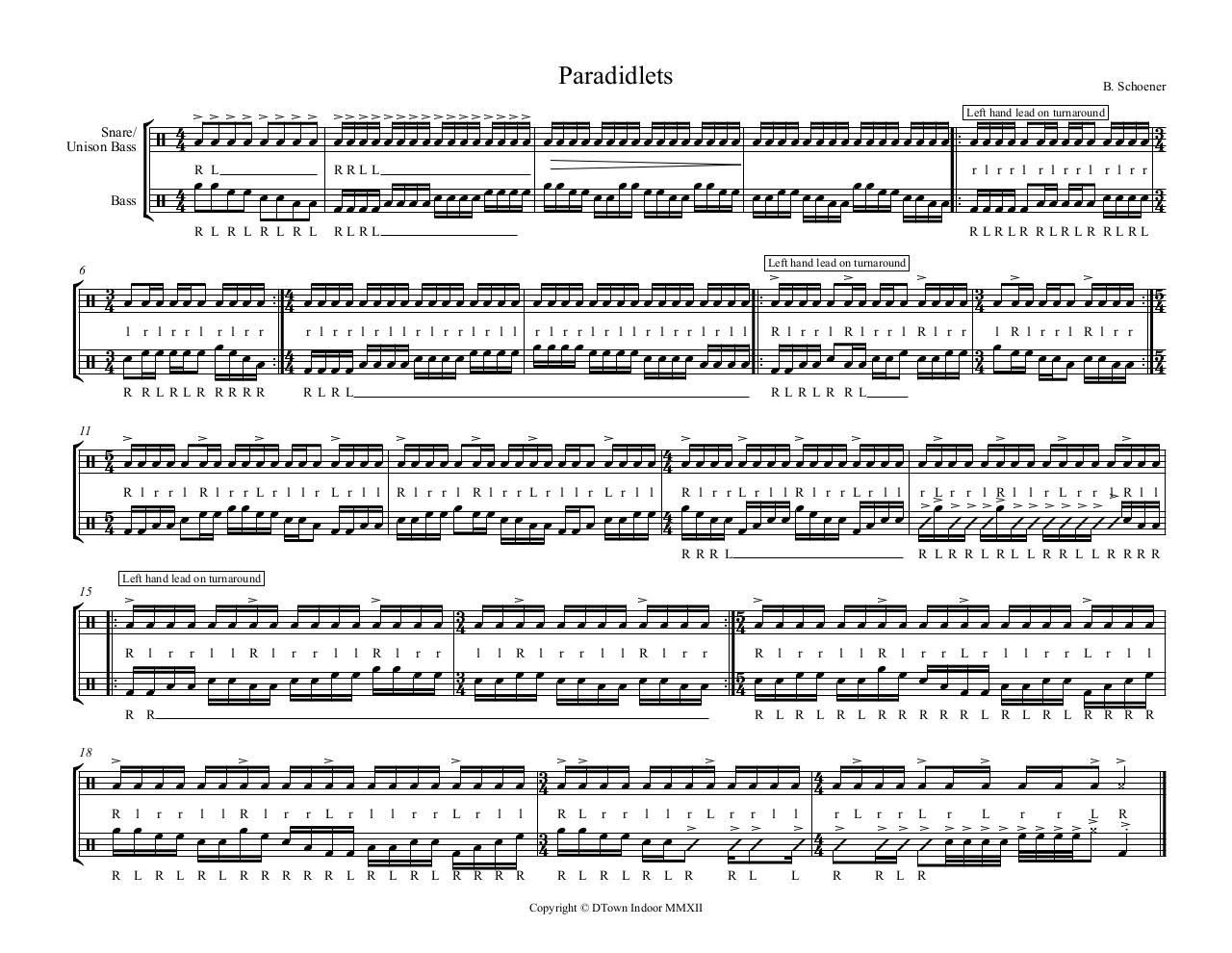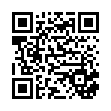### File information

Author: Andrew Szypula

This PDF 1.3 document has been generated by Sibelius 5 / Mac OS X 10.7.2 Quartz PDFContext, and has been sent on pdf-archive.com on 01/12/2011 at 04:20, from IP address 144.26.x.x. The current document download page has been viewed 1030 times.
File size: 66 KB (1 page).
Privacy: public file

### Document preview

Snare/
Unison Bass

B. Schoener

          

                 

R L
RRLL
r l r r l r l r r l r l r r

  


  

                 
Bass  

R L R L R L R L

RLRL

RLRLR RLRLR RLRL

 

     


6

   
 
     

   
 


 
l

r l r r l

r l r r

r l r r l r l l r l r r l r l l

R RLRLR RRRR

r l r r l r l l r l r r l r l l

R l r r l R l r r l R l r r

RLRL

l R l r r l R l r r

RLRLR RL

11

 

                     
R l r r l R l r r L r l l r L r l l R l r r l R l r r L r l l r L r l l
R l r r L r l l R l r r L r l l r 
L r r l 
R l l r L r r l R l l



  




  

RRR L

R L R R L R L L R R L L R RRR

                                                

15

    


       
                      
    

 
   

R

l

r

r

l

l

R

l

r

r

l

l

R

l

r

r

l

l

R

l

r

r

l

l

R

l

r

r

R

R R

l

r

r

l

l

R

l

r

r

 

                                      

        
            

 

l

r

l

l

r

r

L

r

l

l

R L R L R L R R R R R L R L R L R R R R

18

R

L

r

r

l

l

R

l

r

r

L

r

l

l

r

r

L

r

l

l

R L R L R L R R R R R L R L R L R R R R

R L

r

r

l

l

r

R L R L R L R

L

 

r

r

R L

l

 

l

r

L

R

L

 
 

r L
r
L
r
r
L R
            


r

R L R#### HTML Code

Copy the following HTML code to share your document on a Website or Blog

#### QR Code### Related keywords